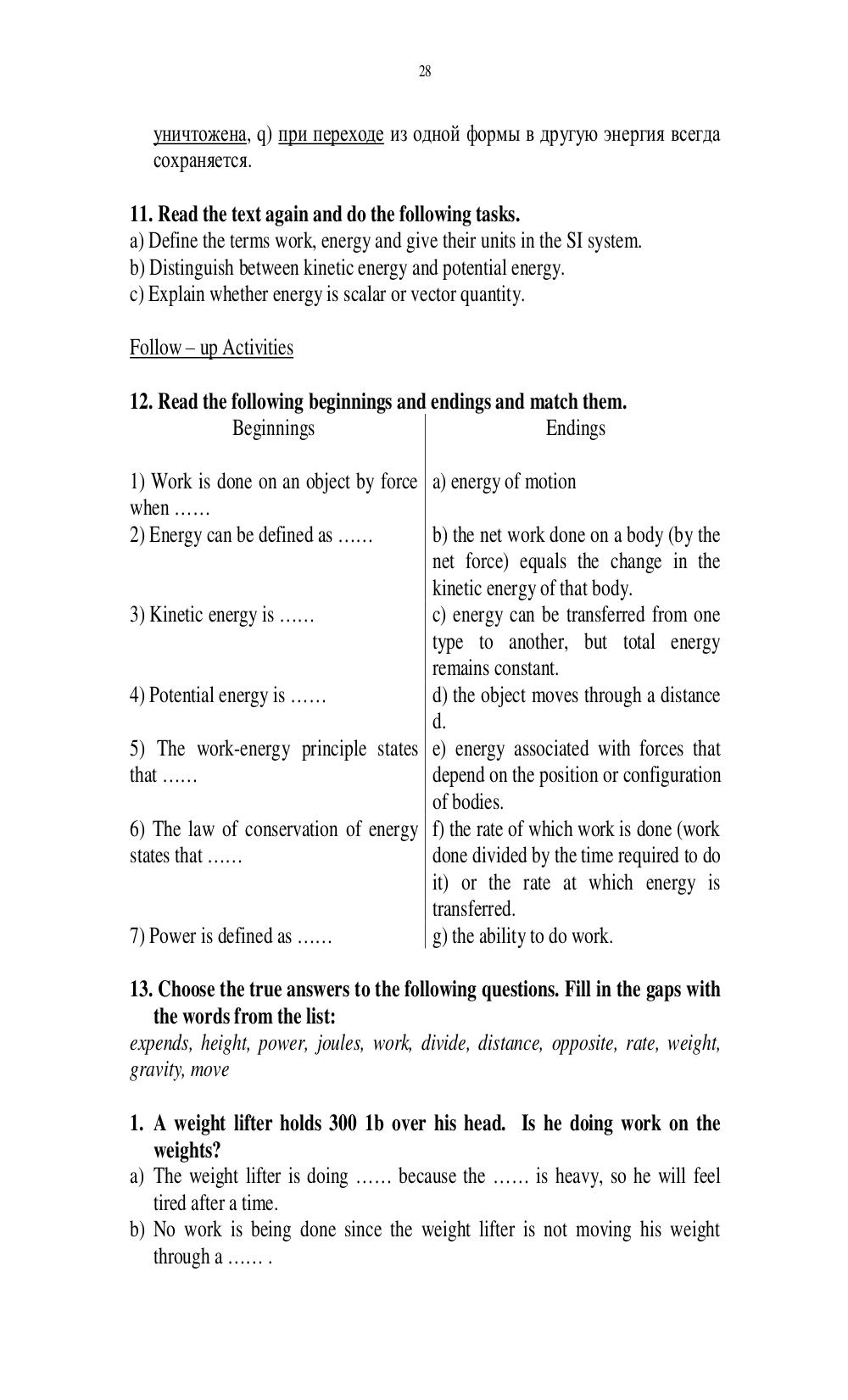# Английский язык. Ильичева Н.А - 28 стр.

Составители:

Рубрика:

• ## Иностранный язык28
уничтожена, q) при переходе из одной формы в другую энергия всегда
сохраняется.
а) Define the terms work, energy and give their units in the SI system.
b) Distinguish between kinetic energy and potential energy.
c) Explain whether energy is scalar or vector quantity.
12. Read the following beginnings and endings and match them.
Beginnings Endings
1) Work is done on an object by force
when ……
a) energy of motion
2) Energy can be defined as …… b) the net work done on a body (by the
net force) equals the change in the
kinetic energy of that body.
3) Kinetic energy is …… c) energy can be transferred from one
type to another, but total energy
remains constant.
4) Potential energy is …… d) the object moves through a distance
d.
5) The work-energy principle states
that ……
e) energy associated with forces that
depend on the position or configuration
of bodies.
6) The law of conservation of energy
states that ……
f) the rate of which work is done (work
done divided by the time required to do
it) or the rate at which energy is
transferred.
7) Power is defined as …… g) the ability to do work.
13. Choose the true answers to the following questions. Fill in the gaps with
the words from the list:
expends, height, power, joules, work, divide, distance, opposite, rate, weight,
gravity, move
1. A weight lifter holds 300 1b over his head. Is he doing work on the
weights?
a) The weight lifter is doing …… because the …… is heavy, so he will feel
tired after a time.
b) No work is being done since the weight lifter is not moving his weight
through a …… .28

уничтожена, q) при переходе из одной формы в другую энергия всегда
сохраняется.

а) Define the terms work, energy and give their units in the SI system.
b) Distinguish between kinetic energy and potential energy.
c) Explain whether energy is scalar or vector quantity.

12. Read the following beginnings and endings and match them.
Beginnings                            Endings

1) Work is done on an object by force a) energy of motion
when ……
2) Energy can be defined as ……        b) the net work done on a body (by the
net force) equals the change in the
kinetic energy of that body.
3) Kinetic energy is ……               c) energy can be transferred from one
type to another, but total energy
remains constant.
4) Potential energy is ……             d) the object moves through a distance
d.
5) The work-energy principle states e) energy associated with forces that
that ……                               depend on the position or configuration
of bodies.
6) The law of conservation of energy f) the rate of which work is done (work
states that ……                        done divided by the time required to do
it) or the rate at which energy is
transferred.
7) Power is defined as ……             g) the ability to do work.

13. Choose the true answers to the following questions. Fill in the gaps with
the words from the list:
expends, height, power, joules, work, divide, distance, opposite, rate, weight,
gravity, move

1. A weight lifter holds 300 1b over his head. Is he doing work on the
weights?
a) The weight lifter is doing …… because the …… is heavy, so he will feel
tired after a time.
b) No work is being done since the weight lifter is not moving his weight
through a …… .